# Math Olympiad Test: Lines and Angles- 2

## 15 Questions MCQ Test Mathematics Olympiad for Class 9 | Math Olympiad Test: Lines and Angles- 2

Description
Attempt Math Olympiad Test: Lines and Angles- 2 | 15 questions in 15 minutes | Mock test for Class 9 preparation | Free important questions MCQ to study Mathematics Olympiad for Class 9 for Class 9 Exam | Download free PDF with solutions
QUESTION: 1

### Two complementary angles are such that two times the measure of one is equal to three times the measure of the other. The measure of the larger angle is:

Solution:

Let the measure of angle be x.
∴ Its complement = (90° - x)
∴  2x = 3(90° - x)
⇒  5x = 3 × 90°
⇒ x = 54°
and  (90° - x ) = 36°
∴ Measure of larger angle = 54°

QUESTION: 2

### In the figure, OA and OB are opposite rays ∠AOC +∠BOD = 63°. The measure of angle ∠COD is;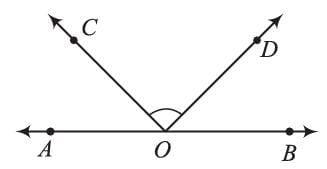Solution:

∵ OA and OB are opposite rays.
∴ ∠AOB is a straight angle.
⇒ ∠AOB = 180°
⇒ (∠AOC + ∠BOD) + ∠COD = 180°
⇒ 63° + ∠COD = 180°
⇒  ∠COD = 117°

QUESTION: 3

### Determine the value of x from the given figure;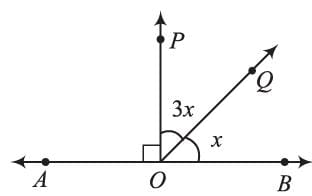Solution:

∠AOB = 180°
⇒ ∠AOP + ∠POB = 180°
⇒ ∠AOP + ∠POQ + ∠BOQ = 180°
⇒ 90° + 3x + x = 180°
⇒  4x = 90°
⇒ x = 90°/4 = 22.5°

QUESTION: 4

b = a + 20°, then a =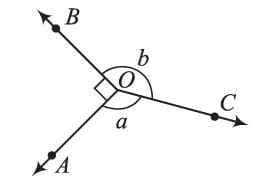Solution:

∵ Sum of angles around a point = 360°
∠AOB + ∠BOC = 360°
⇒ 90° + a + b = 360°
⇒ a + b = 270° ...(i)  and
b = a + 20° ...(ii)
Using (ii) in (i)
a + (a + 20°) = 270°
⇒ 2a = 250°
⇒ a = 125°

QUESTION: 5

The value of m is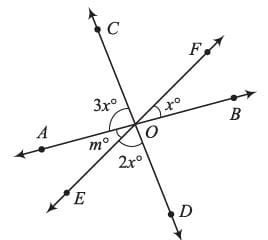Solution:

∠m = ∠x  [Vertically opposite ∠s]
∵ ∠AOB = 180°
⇒ ∠BOF + ∠COF + ∠AOC = 180°
⇒ ∠BOF + ∠DOE +∠AOC = 180°
⇒ x° + 2x° + 3x° = 180°
⇒  6x° = 180°
⇒ x = 30°
∴ m = 30°

QUESTION: 6

Find x from the figure.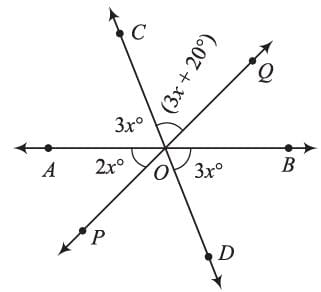Solution:

Here ∠COQ = ∠POD   [vertically opposite ∠s]
∵ ∠AOB = 180°    (AOB is a straight line)
⇒ ∠POA + ∠POD + ∠BOD = 180°
⇒ 2x° +3x° + 20° +3x° = 180°
⇒ 8x = 160°
⇒ x = 20°

QUESTION: 7

x = 3y = 6/7 Z, then, find the value of y.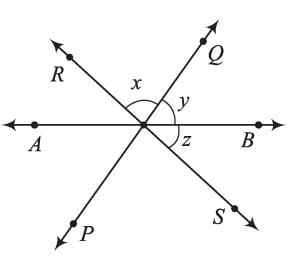Solution:

x = 3y, z = 21/6 y = 7/2y.
∴ x + y + z = 180°
⇒ 3y + y + 7/2 y = 180°
⇒ 4y + 7/2 y = 180°
⇒ 15y = 180° × 2
⇒ y = 24°

QUESTION: 8

Find x from the adjoining figure: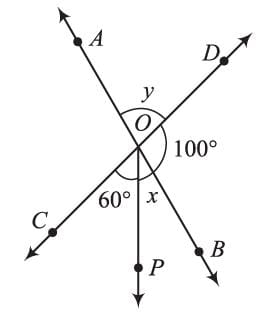Solution:

∠AOD = ∠BOC [vertically opposite ∠s]
⇒ y = 60° + x   ...(i) and,
∵ ∠DOC = 180°
⇒ 60° + x + 100° = 180°
⇒ x = 20°

QUESTION: 9

In the figure, l ∥ m, Find k.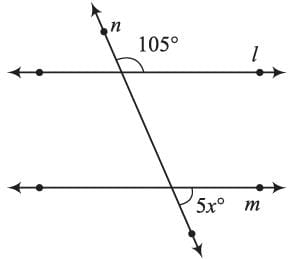Solution:

It is clear from the figure that,
105° + 5x = 180°
⇒   5x = 75°
⇒ x = 15°

QUESTION: 10

AB || CD, and ∠RQB = 115°, and ∠PRQ = 30°. The measure of ∠APC is: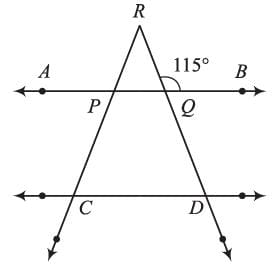Solution:

Here ∠RQB + ∠RQP =180°   (∵ AB is a straight line)
⇒ ∠RQP = 180° - 115° = 65°
Now ∠PRQ = 30°
∵ ∠PRQ, ∠RQP and ∠APQ are the ∠S of D
∴ ∠PRQ + ∠RQP + ∠APQ = 180°
⇒ ∠APQ = 180°- 65°- 30° = 85°
∠APC = ∠APQ [Vertically opposite ∠S]
∴ ∠APC = 85°

QUESTION: 11

If x : y = 2 : 3, then the value of y is equal to: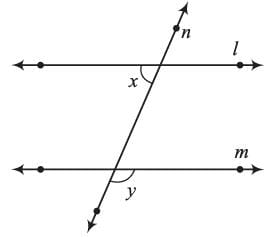Solution:

∵  X : Y = 2 : 3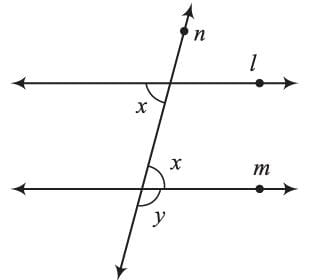∴ Let the angles x and y be 2k and 3k respectively.
∴ 2k + 3k = 180°
[Sum of ∠S in the interior of transversal]
⇒ 5k = 180° ⇒ k = 36°
∴ y = 3k = 3 × 36° = 108°

QUESTION: 12

AB || CD || EF and GH || KL. The measure of ∠HKL is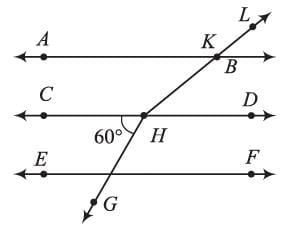Solution:

Extending GH to M, we have,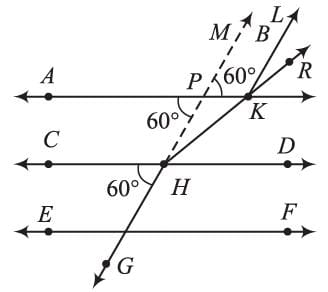∠CHG = ∠APH = 60° [Corresponding ∠S]
∠APH = ∠MPD = 60°  [Vertically opposite ∠S]
∠APH = ∠MPD, then,
∠MPD + ∠LKP = 180° [Sum of interior ∠S]
⇒ ∠LKP = 180° - 60° = 120°, also
∠KHD = ∠PKH = 25° (Alternate ∠S)
∴ ∠HKL = ∠LKP + ∠PKH
= 120° + 25° = 145°

QUESTION: 13

Find x from the given figure (CP || DQ):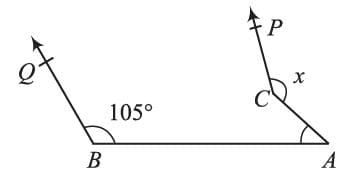Solution:

Construct a line l || AB,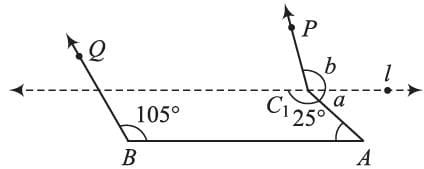∠a = 25° [ Alternate ∠S]
∠c = 105°
∴ ∠b = 105° [Vertically opposite ∠S]
∴ x = a + b = 25° + 105° = 130°

QUESTION: 14

AB || CD and PQ || RS, then x - y =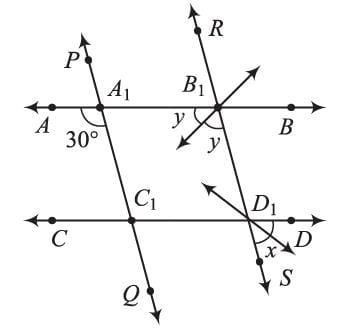Solution:

∠DD1S = ∠B1D1C1 = 2x   [Vertically opposite ∠S]
∠B1D1C1 + ∠A1 B1D1 = 180° [Interior ∠S]
⇒  2x + 2y = 180°
⇒ x + y = 90° ...(i)
Also,
∠AA1C+ ∠A1B1D1  [Corresponding ∠S]
⇒ 30° = 2y
⇒ y = 15° ...(ii)
Using (ii) in (i), we get
x = 90° - y = 90° - 15° = 75°

QUESTION: 15

The value of y, if AB || PQ is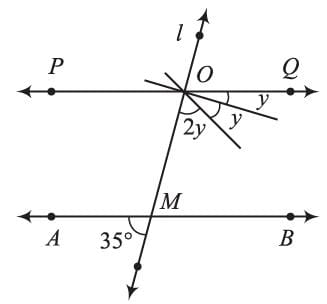Solution:

From the figure,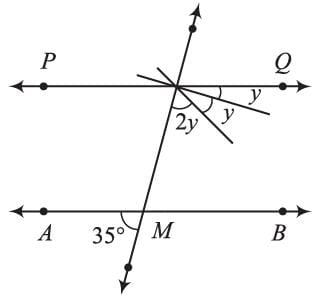(2y + y + y) + 35° = 180° (Interior ∠S)
⇒ 5y = 180° - 35°
⇒ y = 36° - 7
= 29°Use Code STAYHOME200 and get INR 200 additional OFF Use Coupon Code